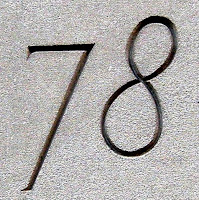## Tuesday, November 2, 2010

### 78

78 = 2 x 3 x 13.

78 has a unique representation as a sum of three squares: 78 = 22 + 52 + 72.

78 is the smallest number with seven representations as a sum of two primes: 78 = 5 + 73 = 7 + 71, 11 + 67 = 17 + 61 = 19 + 59 = 31 + 47 = 37 + 41.

78 is the twelfth triangular number. According to the song "The Twelve Days of Christmas," 78 gifts were sent on the twelfth day.

78 is 66 in base 12.A 78 is an old type of gramophone record playing at 78 revolutions per minute.

Source: Phillips, R. 2004. Numbers: Facts, Figures and Fiction. Badsey Publications.